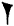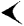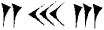# Cuneiform Mathematical Texts

The following article is from The Great Soviet Encyclopedia (1979). It might be outdated or ideologically biased.

## Cuneiform Mathematical Texts

mathematical texts of ancient Babylonia and Assyria, encompassing the period from the beginning of the second millennium B.C. to the beginning of the Common Era. Cuneiform mathematical texts were written in cuneiform on clay tablets. The texts include mathematical tables (such as multiplication tables, tables of reciprocals used to replace division with multiplication, tables of squares and cubes) and special mathematical texts containing problems and solutions. Most of the special texts (of which more than 100 are known) date from the second millennium B.C. Five or six texts of the first millennium B.C. from the Hellenistic era and one text from the Assyrian era have also been found.

Cuneiform mathematical texts have great significance in the history of mathematics since positional notation and quadratic equations are first encountered in them. The Babylonian mathematicians used the sexagesimal number system, in which units were designated byTens were designated byThese symbols were also used to designate units and tens of successive orders; for example, the number

153 = 2 · 60 + 33

was represented as:A unique feature of the Babylonian system of notation was that the absolute value of numbers remained undefined. For example, the number mentioned above could be read as 2 · 602 + 33 · 60 = 9180 and as 2 + 33 · 60”1 = 2 33/60. Moreover, in the texts of the second millennium B.C. there was no symbol corresponding to our zero. If we take into account that the cuneiform mathematical texts have no notations for intermediate calculations, then this phenomenon can be explained most simply by the fact that intermediate calculations were carried out on a counting board (such as our abacus). This also may explain the absence of zero, which is not needed for calculations with the abacus (the column corresponding to a missing digit remained empty). It may be assumed that the appearance of the positional principle was also connected with the use of the counting board.

The Babylonians developed quadratic equations in connection with surveying, a fact reflected in the terminology: unknowns were called “length” and “width.” Subsequently, unknowns were construed more abstractly, and the rudiments of algebraic thought can be found among the Babylonians.

### REFERENCES

Vygodskii, M. Ia. Arifmetika i algebra v drevnem mire, 2nd ed. Moscow, 1967.
Neugebauer, O. Lektsii po istorii antichnykh matematicheskikh nauk, vol. 1. Moscow-Leningrad, 1937. (Translated from German.)
Mathematische Keilschrift-Texte, part 1. Edited by O. von Neugebauer. Berlin, 1935.
Mathematical Cuneiform Texts. Edited by O. Neugebauer and A. Sachs. New Haven, 1945.